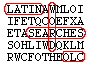## Word Search for Word Forms from OLC Chapter 1

You may wish to print out this exercise.

Read the following sentences and find/circle the Latin words corresponding to the underlined English words. Note that the Latin forms are inflected, so be sure to circle the complete form.

 1. She walks home. 2. Their house is not large. 3. Dinner is on the table. 4. The woman works hard. 5. She is tired. 6. The dog goes into the house. 7. He works in the field. 8. The girl is happy. 9. I'll be home soon. 10. She is prepared. 11. The girl is happy. 12. The dog does not work, but he is still tired.

```x e b f a d c e n a e s
n r q i l m p g n k x b
u r q p z e b d x w n f
e x y u s o v u j r w e
b s c e r k c l l s u m
i e a l c d t a a a o i
k d o l y p m g s e t n
x s f a s h a l w a t a
g f z h t e h r z v d a
d l a b o r a t a m o x
w p d c d n f q c t c m
i n t r a t f e s s a n
```